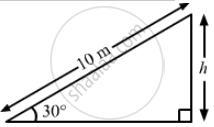Advertisement Remove all ads

# A Block of Mass 5.0 Kg Slides Down an Incline of Inclination 30° and Length 10 M. Find the Work Done by the Force of Gravity. - Physics

Sum

A block of mass 5.0 kg slides down an incline of inclination 30° and length 10 m. Find the work done by the force of gravity.

Advertisement Remove all ads

#### Solution

$\text{ Mass of the block, M = 5 kg }$
$\text{ Angle of inclination } , \theta = 30^\circ$

Gravitational force acting on the block,

$F = mg$
Work done by the force of gravity depends only on the height of the object, not on the path length covered by the object.$\text{ Height of the object , h }= 10 \times \sin30^\circ$
$= 10 \times \frac{1}{2} = 5 m$

$\therefore \text{ Work done by the force of gravity, w = mgh }$

$= 5 \times 9 . 8 \times 5 = 245 J$

Is there an error in this question or solution?
Advertisement Remove all ads

#### APPEARS IN

HC Verma Class 11, 12 Concepts of Physics 1
Chapter 8 Work and Energy
Q 4 | Page 132
Advertisement Remove all ads
Advertisement Remove all ads
Share
Notifications

View all notifications

Forgot password?